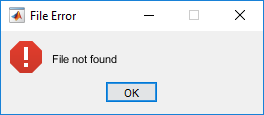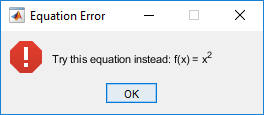errordlg

说明

f = errordlg(msg) 用指定的错误消息创建非模态错误对话框并返回对话框 Figure 对象 f。消息文本会换行以适应对话框大小。

f = errordlg(msg,title) 指定自定义对话框标题。

opts 设置为 'non-modal''modal''replace' 时，f = errordlg(msg,title,opts) 指定窗口样式。如果 opts 是结构体数组，则该函数会为 msg 参数指定窗口样式和解释器。

f = errordlg 创建一个错误对话框，包含如下所示的默认标题和消息。

• 默认标题：错误对话框

• 默认消息：这是默认错误。

示例opts = struct('WindowStyle','modal',...
'Interpreter','tex');
f = errordlg('Try this equation instead: f(x) = x^2',...
'Equation Error', opts);输入参数

• 如果您将错误消息指定为字符向量，则 MATLAB 将对文本进行换行以适应对话框大小。

• 如果您将错误消息指定为元胞数组，则 MATLAB 将在每个元胞数组元素后对文本进行换行。对于较长的元胞数组元素，MATLAB 将对其文本进行换行以适应对话框大小。

'non-modal'

'modal'

'replace'

WindowStyle

'non-modal''modal' 'replace'

Interpreter

'none''tex'。如果设置为 'tex'，则 MATLAB 使用 TeX 解释器来显示消息。

^{ }上标'text^{superscript}'
_{ }下标'text_{subscript}'
\bf粗体'\bf text'
\it斜体'\it text'
\sl伪斜体（通常与斜体相同）'\sl text'
\rm常规字体'\rm text'
\fontname{specifier}字体名称 - 将 specifier 替换为字体系列的名称。您可以将此说明符与其他修饰符结合使用。'\fontname{Courier} text'
\fontsize{specifier}字体大小 - 将 specifier 替换为以磅为单位的数值标量值。'\fontsize{15} text'
\color{specifier}字体颜色 - 将 specifier 替换为以下颜色之一：redgreenyellowmagentablueblackwhitegraydarkGreenorangelightBlue'\color{magenta} text'
\color[rgb]{specifier}自定义字体颜色 - 将 specifier 替换为三元素 RGB 三元组。'\color[rgb]{0,0.5,0.5} text'

\alpha

α

\upsilon

υ

\sim

~

\angle

\phi\leq

\ast

*

\chi

χ

\infty

\beta

β

\psi

ψ

\clubsuit

\gamma

γ

\omega

ω

\diamondsuit

\delta

δ

\Gamma

Γ

\heartsuit

\epsilon

ϵ

\Delta

Δ

\zeta

ζ

\Theta

Θ

\leftrightarrow

\eta

η

\Lambda

Λ

\leftarrow

\theta

θ

\Xi

Ξ

\Leftarrow

\vartheta

ϑ

\Pi

Π

\uparrow

\iota

ι

\Sigma

Σ

\rightarrow

\kappa

κ

\Upsilon

ϒ

\Rightarrow

\lambda

λ

\Phi

Φ

\downarrow

\mu

µ

\Psi

Ψ

\circ

º

\nu

ν

\Omega

Ω

\pm

±

\xi

ξ

\forall

\geq

\pi

π

\exists

\propto

\rho

ρ

\ni

\partial

\sigma

σ

\cong

\bullet

\varsigma

ς

\approx

\div

÷

\tau

τ

\Re

\neq

\equiv

\oplus

\aleph

\Im

\cup

\wp

\otimes

\subseteq

\oslash

\cap

\in

\supseteq

\supset

\lceil

\subset

\int

\cdot

·

\o

ο

\rfloor

\neg

¬

\nabla

\lfloor

\times

x

\ldots

...

\perp

\surd

\prime

´

\wedge

\varpi

ϖ

\0

\rceil

\rangle

\mid

|

\vee

\langle

提示

• 模态对话框（使用 errordlgmsgboxwarndlg 创建）会替代使用这些具有相同名称的函数创建的任何现有对话框。

• 即使模态对话框处于活动状态，MATLAB 程序也会继续执行。要阻止该程序执行直到用户关闭对话框为止，请使用 uiwait 函数。

• 要为单窗口 App 设计工具或基于 uifigure 的 App 创建模态警报对话框，请改用 uialert 函数。